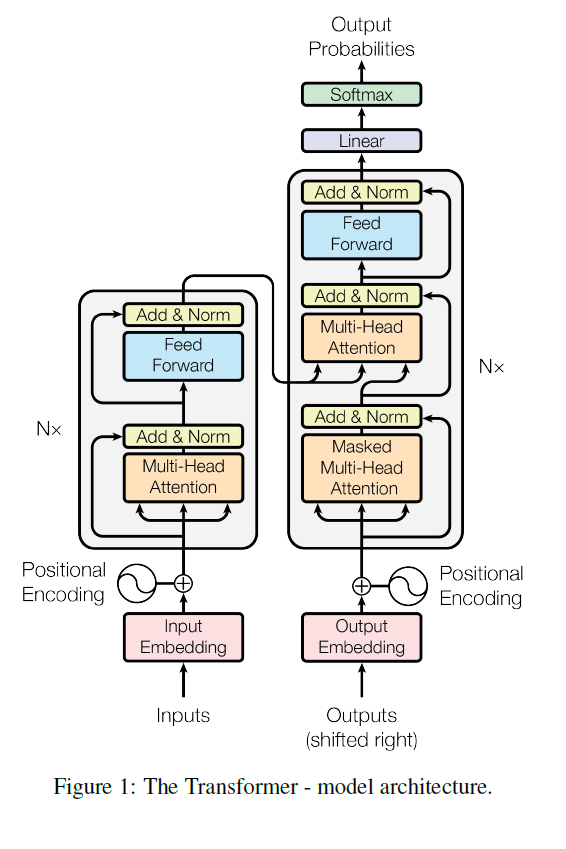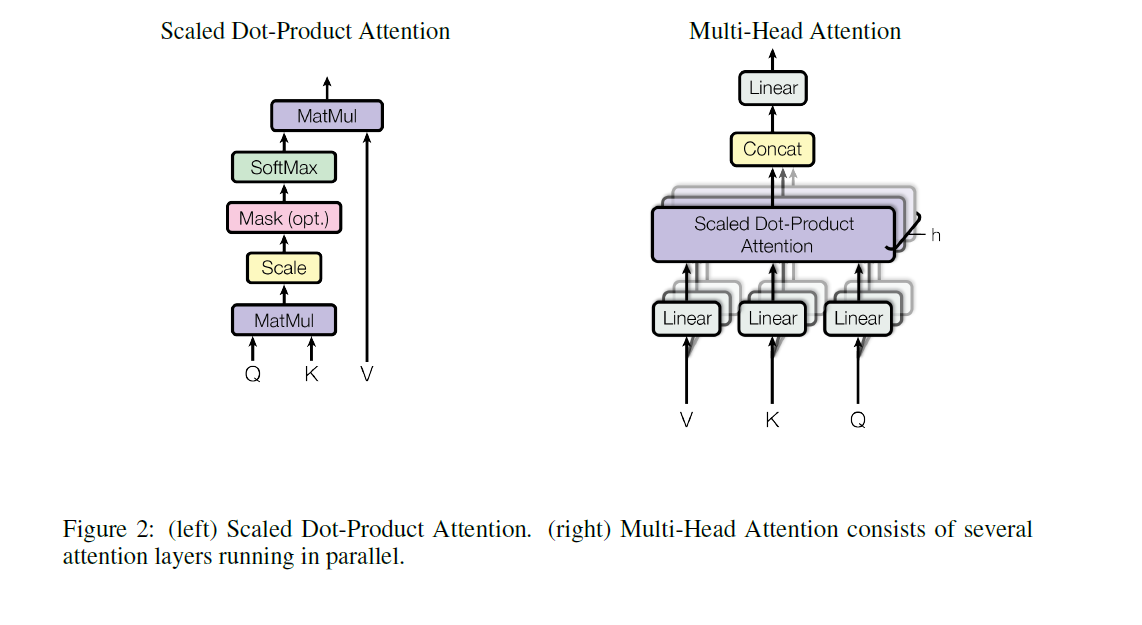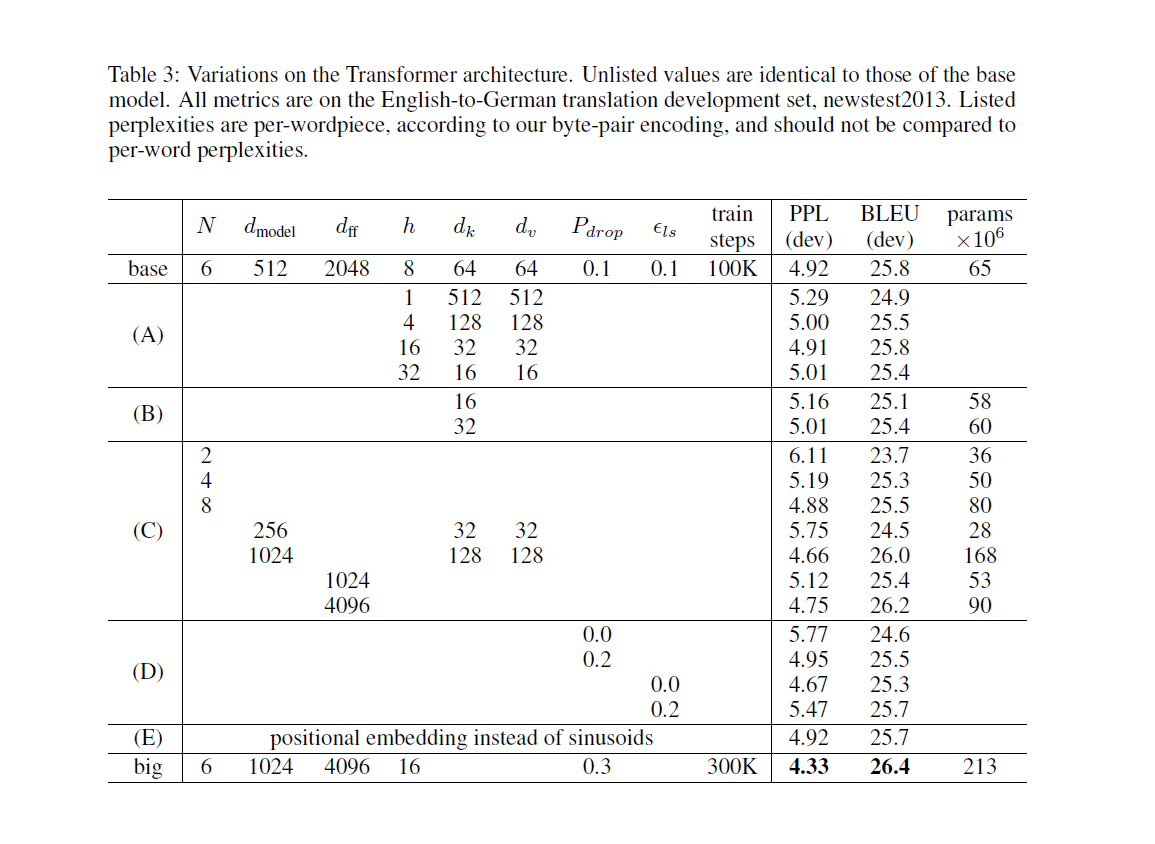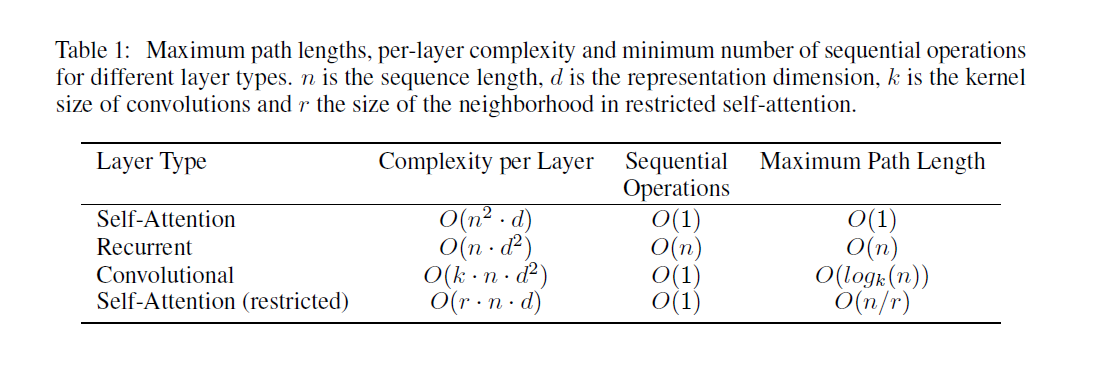﻿ NLP经典论文：Self-attention、Transformer 笔记

### NLP经典论文：Self-attention、Transformer 笔记

2021-12-6 0:15:52

### NLP经典论文：Self-attention、Transformer 笔记

• 论文
• 模型结构
• 整体结构
• 输入
• 输出
• 文章部分翻译
• 3 Model Architecture
• 3.1 Encoder and Decoder Stacks
• 3.2 Attention
• 3.2.1 Scaled Dot-Product Attention
• 3.2.3 Applications of Attention in our Model
• 3.3 Position-wise Feed-Forward Networks
• 3.4 Embeddings and Softmax
• 3.5 Positional Encoding
• 4 Why Self-Attention
• 相关视频
• 相关的笔记
• 相关代码
• pytorch
• tensorflow
• keras
• pytorch API:
• tensorflow API

NLP论文笔记合集（持续更新）

# 模型结构

## 整体结构### 输入

x = ( x 1 , … , x n ) \mathbf{x}=(x_1,…,x_n) x x 为一个中文词， x \mathbf{x} 为中文的一个句子。

### 输出

y = ( y 1 , … , y m ) \mathbf{y}=(y_1,…,y_m) y y 为一个英文词， x \mathbf{x} 为英文的一个句子。

# 文章部分翻译

## 3 Model Architecture

Transformer 遵循这一总体架构，encoder和decoder都使用堆叠的self-attention和逐点全连接层，分别如图1的左半部分和右半部分所示。### 3.1 Encoder and Decoder Stacks

E n c o d e r \boldsymbol{\mathbf{Encoder}} ：encoder由N=6个相同层堆叠而成。每层有两个子层。第一个子层是multi-head self-attention结构，第二个子层是简单的、对应位置逐个相乘的全连接前馈网络。我们在两个子层的每个层周围使用residual connection，然后进行layer normalization。也就是说，每个子层的输出是LayerNorm（x+Sublayer（x）），其中Sublayer（x）是由子层本身实现的函数。为了便于residual connection进行连接，模型中的所有子层以及embedding层都会生成尺寸为 d m o d e l = 512 d_{model}=512 的输出。

D e c o d e r \boldsymbol{\mathbf{Decoder}} ：decoder也由N=6个相同层的堆栈组成。除了每个encoder层中的两个子层之外，decoder还插入第三个子层，该子层对encoder堆的输出执行multi-head attention。与encoder类似，我们在每个子层周围使用residual connection，然后进行layer normalization。我们还修改了decoder堆栈中的self-attention子层，以防止当前位置关注到未来位置的信息。这种masking，基于输出的embedding偏移一个位置的事实，确保位置 i i 的预测只能依赖于位置小于 i i 的已知输出。

### 3.2 Attention

attention函数可以描述为将query和一组key-value对映射到输出的一个映射，其中query、key、value和输出都是向量。输出由value的加权计算得到，其中分配给每个value的权重由query的匹配函数与相应key计算得到。#### 3.2.1 Scaled Dot-Product Attention

A t t e n t i o n ( Q , K , V ) = s o f t m a x ( Q K T d k ) V Attention(Q,K,V)=softmax(\frac{QK^T}{\sqrt{d_k}})V

4 ^4 为了说明点积变大的原因，假设组成元素 q q k k 的平均值为0，方差为1的独立随机变量。那么它们的点积 q ⋅ k = ∑ i = 1 d k q i k i q\cdot k=\sum_{i=1}^{d_k} q_ik_i 的均值为0，方差为 d k d_k

M u l t i H e a d ( Q , K , V ) = C o n c a t ( h e a d 1 , . . . , h e a d h ) W O MultiHead(Q, K, V ) = Concat(head_1, ..., head_h)W^O w h e r e h e a d i = A t t e n t i o n ( Q W i Q , K W i K , V W i V ) where\quad head_i = Attention(QW_i^Q, KW_i^K , VW_i^V)

#### 3.2.3 Applications of Attention in our Model

•在“encoder-decoder attention”层中，query来自前一个decoder子层，key和value来自encoder的输出。这允许decoder中的每个位置都关注到输入序列的所有位置。这模仿了sequence-to-sequence模型中典型的encoder-decoder attention机制，如[31,2,8]。

•encoder包含self-attention层。在self-attention层中，所有query、key和value都来自同一个位置，在本例中，是encoder中前一层的输出。encoder中的每个位置都可以关注encoder前一层中的所有位置。

•类似地，decoder中的self-attention层允许decoder中的每个位置关注decoder中直到并包括该位置的所有位置。我们需要防止decoder中的信息向左流动，以保持自回归特性。在scaled dot-product attention中，我们通过mask softmax输入中，所有与非法连接对应的值（设置为 − ∞ \infty )，来实现这一点 。参见图2。

### 3.3 Position-wise Feed-Forward Networks

F F N ( x ) = max ⁡ ( 0 , x W 1 + b 1 ) W 2 + b 2 FFN(x) = \max(0, xW_1 + b_1)W_2 + b_2

### 3.4 Embeddings and Softmax

（注：感觉这里共享是因为，中文和英文的词，意思相同的话，都会在word embedding过程中映射到同一个位置。）

### 3.5 Positional Encoding

P E ( p o s , 2 i ) = s i n ( p o s / 1000 0 2 i / d m o d e l ) PE_{(pos,2i)} = sin(pos/10000^{2i/d_{model}}) P E ( p o s , 2 i + 1 ) = c o s ( p o s / 1000 0 2 i / d m o d e l ) PE_{(pos,2i+1)} = cos(pos/10000^{2i/d_{model}})## 4 Why Self-Attention# 相关视频

Transformer论文逐段精读【论文精读】

Sequence-to-sequence Learning
【李宏毅】Transformer Seq2seq

# 相关代码

## pytorch

MorvanZhou /NLP-Tutorials：代码中n代表，即包含batch size；step代表输入句子长度，即一句话包含多少个词；model_dim、dim、emb_dim代表word embedding的维度。